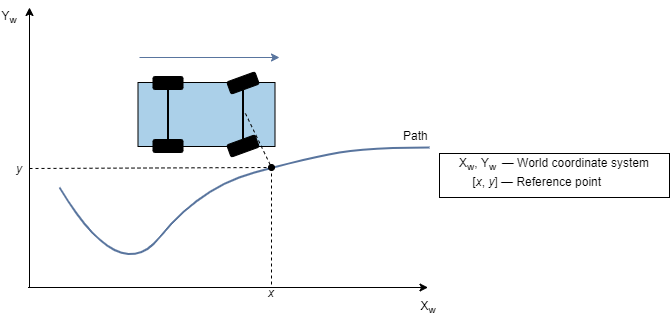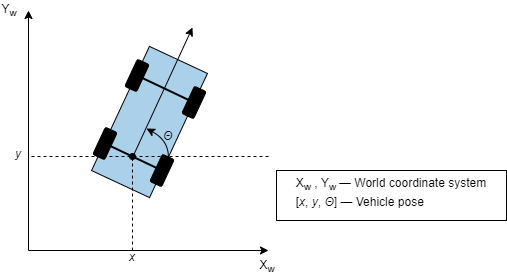# lateralControllerStanley

Compute steering angle command for path following by using Stanley method

Since R2018b

## Syntax

``steerCmd = lateralControllerStanley(refPose,currPose,currVelocity)``
``steerCmd = lateralControllerStanley(refPose,currPose,currVelocity,Name,Value)``

## Description

example

````steerCmd = lateralControllerStanley(refPose,currPose,currVelocity)` computes the steering angle command, in degrees, that adjusts the current pose of a vehicle to match a reference pose, given the current velocity of the vehicle. By default, the function assumes that the vehicle is in forward motion.The controller computes the steering angle command using the Stanley method , whose control law is based on a kinematic bicycle model. Use this controller for path following in low-speed environments, where inertial effects are minimal.```

example

````steerCmd = lateralControllerStanley(refPose,currPose,currVelocity,Name,Value)` specifies options using one or more name-value pairs. For example, `lateralControllerStanley(refPose,currPose,currVelocity,'Direction',-1)` computes the steering angle command for a vehicle in reverse motion.```

## Examples

collapse all

Compute the steering angle command that adjusts the current pose of a vehicle to a reference pose along a driving path. The vehicle is in forward motion.

In this example, you compute a single steering angle command. In path-following algorithms, compute the steering angle continuously as the pose and velocity of the vehicle change.

Set a reference pose on the path. The pose is at position (4.8 m, 6.5 m) and has an orientation angle of 2 degrees.

`refPose = [4.8, 6.5, 2]; % [meters, meters, degrees]`

Set the current pose of the vehicle. The pose is at position (2 m, 6.5 m) and has an orientation angle of 0 degrees. Set the current velocity of the vehicle to 2 meters per second.

```currPose = [2, 6.5, 0]; % [meters, meters, degrees] currVelocity = 2; % meters per second```

Compute the steering angle command. For the vehicle to match the reference pose, the steering wheel must turn 2 degrees counterclockwise.

`steerCmd = lateralControllerStanley(refPose,currPose,currVelocity)`
```steerCmd = 2.0000 ```

Compute the steering angle command that adjusts the current pose of a vehicle to a reference pose along a driving path. The vehicle is in reverse motion.

In this example, you compute a single steering angle command. In path-following algorithms, compute the steering angle continuously as the pose and velocity of the vehicle change.

Set a reference pose on the path. The pose is at position (5 m, 9 m) and has an orientation angle of 90 degrees.

`refPose = [5, 9, 90]; % [meters, meters, degrees]`

Set the current pose of the vehicle. The pose is at position (5 m, 10 m) and has an orientation angle of 75 degrees.

`currPose = [5, 10, 75]; % [meters, meters, degrees]`

Set the current velocity of the vehicle to –2 meters per second. Because the vehicle is in reverse motion, the velocity must be negative.

`currVelocity = -2; % meters per second`

Compute the steering angle command. For the vehicle to match the reference pose, the steering wheel must turn 15 degrees clockwise.

`steerCmd = lateralControllerStanley(refPose,currPose,currVelocity,'Direction',-1)`
```steerCmd = -15.0000 ```

## Input Arguments

collapse all

Reference pose, specified as an [x, y, Θ] vector. x and y are in meters, and Θ is in degrees.

x and y specify the reference point to steer the vehicle toward. Θ specifies the orientation angle of the path at this reference point and is positive in the counterclockwise direction.

• For a vehicle in forward motion, the reference point is the point on the path that is closest to the center of the vehicle's front axle.• For a vehicle in reverse motion, the reference point is the point on the path that is closest to the center of the vehicle's rear axle.Data Types: `single` | `double`

Current pose of the vehicle, specified as an [x, y, Θ] vector. x and y are in meters, and Θ is in degrees.

x and y specify the location of the vehicle, which is defined as the center of the vehicle's rear axle.

Θ specifies the orientation angle of the vehicle at location (x,y) and is positive in the counterclockwise direction.For more details on vehicle pose, see Coordinate Systems in Automated Driving Toolbox.

Data Types: `single` | `double`

Current longitudinal velocity of the vehicle, specified as a real scalar. Units are in meters per second.

• If the vehicle is in forward motion, then this value must be greater than 0.

• If the vehicle is in reverse motion, then this value must be less than 0.

• A value of 0 represents a vehicle that is not in motion.

Data Types: `single` | `double`

### Name-Value Arguments

Specify optional pairs of arguments as `Name1=Value1,...,NameN=ValueN`, where `Name` is the argument name and `Value` is the corresponding value. Name-value arguments must appear after other arguments, but the order of the pairs does not matter.

Before R2021a, use commas to separate each name and value, and enclose `Name` in quotes.

Example: `'MaxSteeringAngle',25`

Driving direction of the vehicle, specified as the comma-separated pair consisting of `'Direction'` and either `1` for forward motion or `-1` for reverse motion. The driving direction determines the position error and angle error used to compute the steering angle command. For more details, see Algorithms.

Position gain of the vehicle, specified as the comma-separated pair consisting of `'PositionGain'` and a positive real scalar. This value determines how much the position error affects the steering angle. Typical values are in the range [1, 5]. Increase this value to increase the magnitude of the steering angle.

Distance between the front and rear axles of the vehicle, in meters, specified as the comma-separated pair consisting of `'Wheelbase'` and a real scalar. This value applies only when the vehicle is in forward motion.

Maximum allowed steering angle of the vehicle, in degrees, specified as the comma-separated pair consisting of `'MaxSteeringAngle'` and a real scalar in the range (0, 180).

The `steerCmd` value is saturated to the range `[-MaxSteeringAngle, MaxSteeringAngle]`.

• Values below `-MaxSteeringAngle` are set to `-MaxSteeringAngle`.

• Values above `MaxSteeringAngle` are set to `MaxSteeringAngle`.

## Output Arguments

collapse all

Steering angle command, in degrees, returned as a real scalar. This value is positive in the counterclockwise direction.For more details, see Coordinate Systems in Automated Driving Toolbox.

## Algorithms

To compute the steering angle command, the controller minimizes the position error and the angle error of the current pose with respect to the reference pose. The driving direction of the vehicle determines these error values.

When the vehicle is in forward motion (`'Direction'` name-value pair is `1`):

• The position error is the lateral distance from the center of the front axle to the reference point on the path.

• The angle error is the angle of the front wheel with respect to reference path.

When the vehicle is in reverse motion (`'Direction'` name-value pair is `-1`):

• The position error is the lateral distance from the center of the rear axle to the reference point on the path.

• The angle error is the angle of the rear wheel with respect to reference path.

For details on how the controller minimizes these errors, see .

 Hoffmann, Gabriel M., Claire J. Tomlin, Michael Montemerlo, and Sebastian Thrun. "Autonomous Automobile Trajectory Tracking for Off-Road Driving: Controller Design, Experimental Validation and Racing." American Control Conference. 2007, pp. 2296–2301. doi:10.1109/ACC.2007.4282788